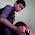# Voltage divider circuit

Voltage divider circuit is also known as potential divider circuit. Input to a voltage divider circuit is Vin.

Vin voltage will drive current (I) through both resistors. Because of both resistors connected in series, then the same current will flows pass through each resistor.Effective resistance of two series resistors are R1 + R2. Drop voltage across this combination of both resistor is a Vin, according to Ohm's Law, the current flowing is
I = Vin / (R1 + R2)
Voltage at R2 becomes
Vout = I x R2
Substituting the current (I) with the first equation
Vout = Vin x R2 / (R1 + R2)

This equation is an equation to calculate the output voltage generated by a voltage divider circuit. By selecting two resistor with resistance values suitable, we can get the value of any output voltage within the range 0 to Vin (Volt).

Next→
←Prev

### Choose label

1.